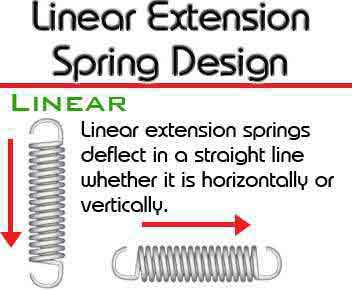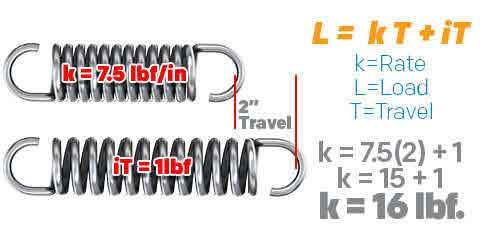<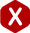# Linear Spring Design

#### Definition: The process of building a design for a linear coil spring made from helically wound round wire.

Linear springs are helical springs with a linear constant force. This means that it’ll take a proportional amount of load for the spring to deflect a certain distance of travel. Linear springs are compression springs and extension springs. What differs these types of springs from a torsion spring, is that torsion springs exert a radial force. Compression and extension springs exert a constant amount of force per every inch or millimeter of distance traveled (fraction of an inch or millimeter as well). The amount of load it’ll take a spring to travel a required distance depends on the spring constant, also known as spring rate or spring force. The difference between a linear compression spring and a linear extension spring is not only the differences in their physical form or how one is used to compress (be pushed on) while the other is used to extend (be pulled on). The difference is that the working loads on a compression spring are only based on spring rate while the constant forces for an extension spring are also based on initial tension.

### Linear Compression Springs

Compression springs deflect in a straight line, whether it might be vertical or horizontal. The amount of force they exert is based on physical dimensions like the wire diameter, outer diameter, free length, active coils (space in between active coils), and the material type used. Some springs may be very similar in shape or size but the force they exert and their elasticity depends on the proportion of all of the dimensions that make up the linear spring. This is due to stress factors like spring index or the proportion within the amount of coils (or space in between these coils) and the free length. If your spring has a tight index (a large wire diameter in proportion to the outer diameter), it is going to be under stress so it will be able to carry a significant load but might not be able to travel (or deflect) much. The same goes for when your spring has a long free length with very few coils. The opposite of these given examples (large index or many coils in proportion to the free length) gives you the opposite reaction because it’ll be under less stress: the spring will be able to deflect much more but it will not be very strong. The following formulas and examples will demonstrate how this works.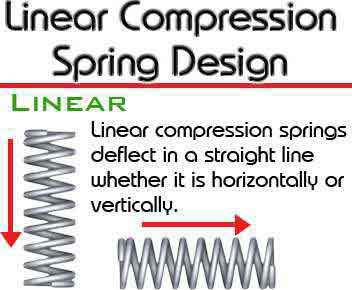#### Spring Index

(This formula works for both compression and extension springs)

Spring index is the proportional ratio between your spring’s diameter and its wire diameter. To calculate spring index, you must subtract one wire diameter from the outer diameter. The result is what we call the mean diameter as shown in the diagrams. Then, you must divide the mean diameter by the wire diameter. The outcome of this calculation is your spring’s index. A 4:1 index is too tight while a 15:1 index is too loose so you want to make sure that you’re somewhere in between.

##### Formula for Spring Index:
Index = (Outer Diameter – Wire Diameter) ÷ Wire Diameter
I = (OD – WD) ÷ WD

Example: You have an outer diameter of 0.5” and a wire diameter of 0.05”.

I = (0.5 – 0.05) ÷ 0.05
I = 0.45 ÷ 0.05
I = 9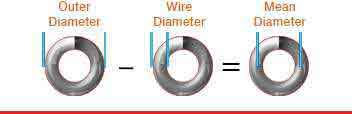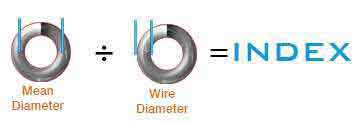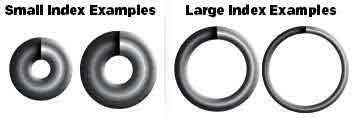#### Spring Rate

##### Formula for Spring Rate:
k = L ÷ T

k = 15 ÷ 2
k = 7.5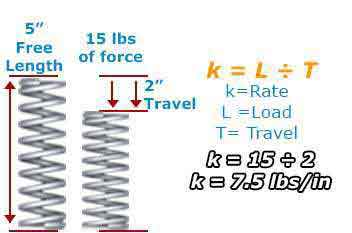### Linear Extension Springs